Test: Functions Of One,Two Or Three Real Variables -1

# Test: Functions Of One,Two Or Three Real Variables -1

Test Description

## 20 Questions MCQ Test Topic-wise Tests & Solved Examples for IIT JAM Mathematics | Test: Functions Of One,Two Or Three Real Variables -1

Test: Functions Of One,Two Or Three Real Variables -1 for IIT JAM 2023 is part of Topic-wise Tests & Solved Examples for IIT JAM Mathematics preparation. The Test: Functions Of One,Two Or Three Real Variables -1 questions and answers have been prepared according to the IIT JAM exam syllabus.The Test: Functions Of One,Two Or Three Real Variables -1 MCQs are made for IIT JAM 2023 Exam. Find important definitions, questions, notes, meanings, examples, exercises, MCQs and online tests for Test: Functions Of One,Two Or Three Real Variables -1 below.
Solutions of Test: Functions Of One,Two Or Three Real Variables -1 questions in English are available as part of our Topic-wise Tests & Solved Examples for IIT JAM Mathematics for IIT JAM & Test: Functions Of One,Two Or Three Real Variables -1 solutions in Hindi for Topic-wise Tests & Solved Examples for IIT JAM Mathematics course. Download more important topics, notes, lectures and mock test series for IIT JAM Exam by signing up for free. Attempt Test: Functions Of One,Two Or Three Real Variables -1 | 20 questions in 60 minutes | Mock test for IIT JAM preparation | Free important questions MCQ to study Topic-wise Tests & Solved Examples for IIT JAM Mathematics for IIT JAM Exam | Download free PDF with solutions
 1 Crore+ students have signed up on EduRev. Have you?
Test: Functions Of One,Two Or Three Real Variables -1 - Question 1

### If f(x) is differentiable on interval l and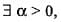such that |f'(x)| ≤ a on l, then f(x) is

Detailed Solution for Test: Functions Of One,Two Or Three Real Variables -1 - Question 1

For xy ∈ l, by Lagrange’s mean value theorem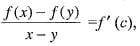where x < c < y
implies f(x)- f(y) = (x - y) f '(c )
implies |f(x) - f(y) | = x - y || f'(c)|
for a given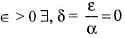such that |f(x) - f(y)| < ε, x, y ∈ l. Hence f(x) is uniformly continuous on l. We know that every uniformly continuous function is also continuous.

Test: Functions Of One,Two Or Three Real Variables -1 - Question 2

### The value of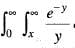dydx by changing the order of integration is

Test: Functions Of One,Two Or Three Real Variables -1 - Question 3

### If f(x) is differentiable on (a, b) and f(a) = 0 and there exists a real number k such that |f'(x) ≤ k |f (x)| on [a, b], then f(x) is

Detailed Solution for Test: Functions Of One,Two Or Three Real Variables -1 - Question 3

Choose x> a such that k(x1 - a) < 1,
let α = sup | f(t) | on a < t < x1 then by Lagrange’s mean value theorem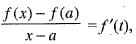where a < t< x ≤ x1
implies f(x) - f(a) = (x - a) f '(t)
[since f(a) = 0]

Test: Functions Of One,Two Or Three Real Variables -1 - Question 4

The volume of ellipsoide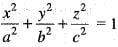is

Test: Functions Of One,Two Or Three Real Variables -1 - Question 5

If f(x+y) = f (x) . f(y) for all x and y. Suppose that f(3) = 3 and f'(0) = 11 then , f'(3) is equal to

Detailed Solution for Test: Functions Of One,Two Or Three Real Variables -1 - Question 5

Given that f'(0) = 11
implies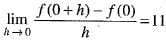implies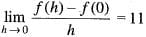implies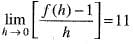[Since f(3 + 0) = f(3) - f(0)
=> f(3) = f(3) • (0)]
implies f(0) = 1 Now we have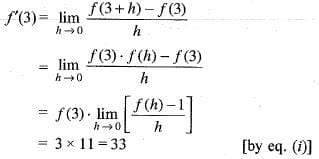Test: Functions Of One,Two Or Three Real Variables -1 - Question 6

The area bounded by the curve y = ψ(x), x-axis and the lines x = l , x = m(l <m ) is given by

Test: Functions Of One,Two Or Three Real Variables -1 - Question 7

If f(x) and g(x) are real number function defined on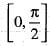and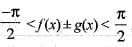, , then which of following is correct?

Detailed Solution for Test: Functions Of One,Two Or Three Real Variables -1 - Question 7

Here,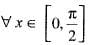cos f(x) - sin (g(x))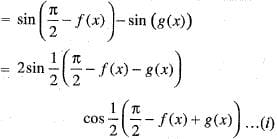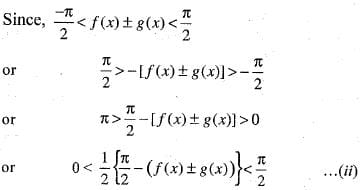using eq. (ii) in eq. (i), then we obtain cos (f (x)) - sin (g (x)) > 0
or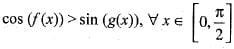Test: Functions Of One,Two Or Three Real Variables -1 - Question 8

The volume of an object expressed in spherical coordinates is given by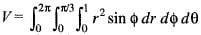The value of the integral is

Test: Functions Of One,Two Or Three Real Variables -1 - Question 9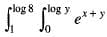dx dy is equal to

Test: Functions Of One,Two Or Three Real Variables -1 - Question 10

Using the transformation x + y = u, y = v. The value of Jacobian (J) for the integral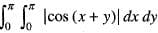is

Test: Functions Of One,Two Or Three Real Variables -1 - Question 11

Which of the following function is not called the Euler’s integral of the first kind?

Detailed Solution for Test: Functions Of One,Two Or Three Real Variables -1 - Question 11

Euler’s integral of the first kind is nothing but Beta function. So, here only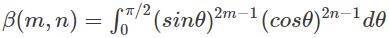is not the definition of Beta function.

Test: Functions Of One,Two Or Three Real Variables -1 - Question 12

Consider the following conditions
(i) f(x) is well defined at x = a
(ii)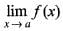must exist.
(iii) f(x) is continuous.
(iv) f(x) ≠ 0 at x = a
Q. which of these conditions are necessary for a function f(x) to be derivable at a point x = a of its domain?

Detailed Solution for Test: Functions Of One,Two Or Three Real Variables -1 - Question 12

The necessary condition for a function f(x) to be derivable at a point x = a of its domain.
(i) f(x) is well defined at x = a
(ii)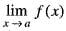must exist.
(iv) f (x) is continuous.

Test: Functions Of One,Two Or Three Real Variables -1 - Question 13

For (x, y) ∈ R2, let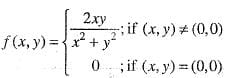Detailed Solution for Test: Functions Of One,Two Or Three Real Variables -1 - Question 13

Let us approaches (0, 0) along the line y = mx which passes through origin. Put y = mx, we get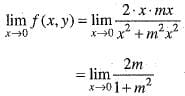which depends on m. Hence,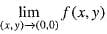does not exists, Therefore f(x, y) is discontinuous at (0,0).
Now,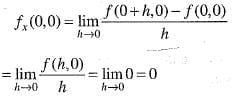and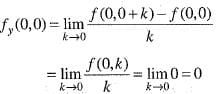Hence, f(x,y) is discontinuous at (0, 0). But both the partial derivative fx and fy exists at origin.

Test: Functions Of One,Two Or Three Real Variables -1 - Question 14

Let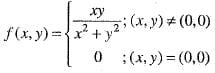Then,

Detailed Solution for Test: Functions Of One,Two Or Three Real Variables -1 - Question 14

Let us suppose (x, y) approaches (0, 0) along the line y = mx. Which is a line through the origin. Put y = mx and allows x —> 0, we get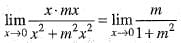which depends on m, therefore the limit of f(x, y) at (0, 0) does not exists. Hence, f(x, y) is discontinuous at origin.
Now,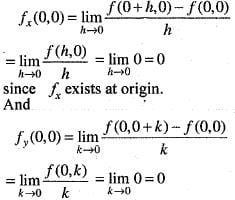since fy exists at origin.

Test: Functions Of One,Two Or Three Real Variables -1 - Question 15

Let f : R2 —> R be defined by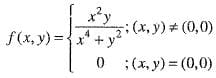Then, the directional derivative direction of f at (0 , 0) in the direction o f the vector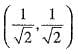is

Detailed Solution for Test: Functions Of One,Two Or Three Real Variables -1 - Question 15

we are given that u =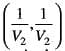and a = (0,0). we have directional derivative at a in the direction u as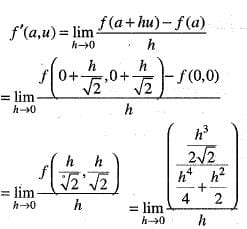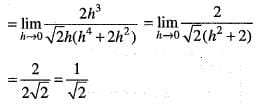Test: Functions Of One,Two Or Three Real Variables -1 - Question 16

L et f : R2 —> R be defined by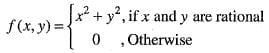Then,

Detailed Solution for Test: Functions Of One,Two Or Three Real Variables -1 - Question 16

We have,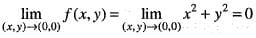But f(0 , 0) = 0
Hence,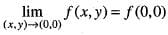implies f(x, y) is continuous at (0, 0) Now,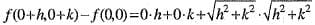where A = 0, B = 0 which does not depends on h and k and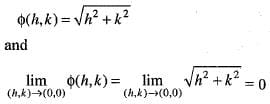Hence, y(x, y) is continuous as well as differentiable at (0, 0).

Test: Functions Of One,Two Or Three Real Variables -1 - Question 17

The inverse of function f(x) = x3 + 2 is ____________

Detailed Solution for Test: Functions Of One,Two Or Three Real Variables -1 - Question 17

To find the inverse of the function equate f(x) then find the value of x in terms of y such that f -1 (y) = x.

Test: Functions Of One,Two Or Three Real Variables -1 - Question 18

Let f : R2 —> R be such that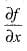and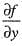exist at all points. Then,

Test: Functions Of One,Two Or Three Real Variables -1 - Question 19

Let if possible,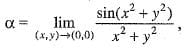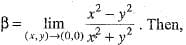Test: Functions Of One,Two Or Three Real Variables -1 - Question 20

Let f: R2 → R be defined by f(x, y)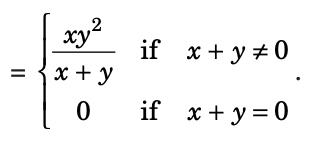Then the value of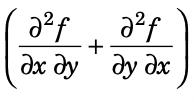at the point (0, 0) is

Detailed Solution for Test: Functions Of One,Two Or Three Real Variables -1 - Question 20

Here, f(x, y)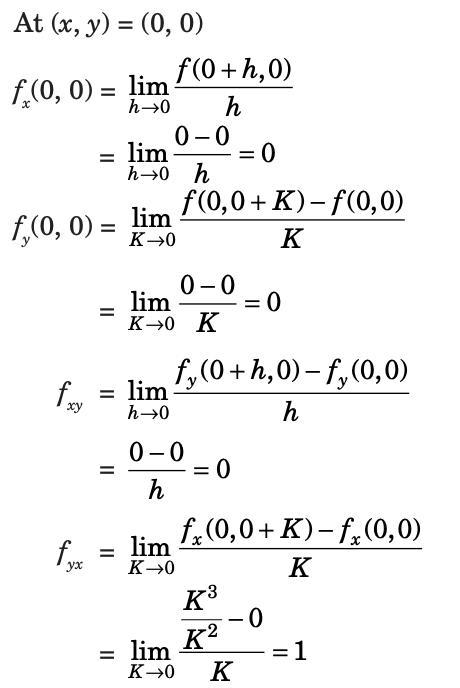## Topic-wise Tests & Solved Examples for IIT JAM Mathematics

27 docs|150 tests
Information about Test: Functions Of One,Two Or Three Real Variables -1 Page
In this test you can find the Exam questions for Test: Functions Of One,Two Or Three Real Variables -1 solved & explained in the simplest way possible. Besides giving Questions and answers for Test: Functions Of One,Two Or Three Real Variables -1 , EduRev gives you an ample number of Online tests for practice

## Topic-wise Tests & Solved Examples for IIT JAM Mathematics

27 docs|150 tests(Scan QR code)